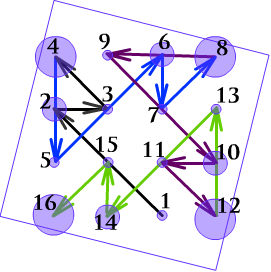# Primitive Modular Quadratics. Part I

Algebra Level 4$\text{Let} : \quad \begin{cases} \quad f(x)=x^2-6x-16 \\ \ \ \ \ g(x)=f(|x|) \\ \ \ \ \ h(x)=|g(x)| \end{cases}$

Find the sum of all real roots of the equation $g(x)=0$.

Author's Note : What you observe in the picture attached to this problem?

×